# Centre of Mass

• Last Updated : 30 Jun, 2021

The Centre of mass is a very integral part of mechanics. It allows us to perform calculations on big bodies by considering them as point objects. These simplifications then became the basis for the development of the different fields of mechanics. For example, rotational mechanics, gravitational mechanics etc. The Centre of mass intuitively means the point from which we can support the object from below. For example, a stick can be supported from a middle point such that it does not fall down. Similarly, every object has a similar point. This is called the Centre of mass

### Centre of Mass

A lot of problems can be simplified if it is assumed that the mass of the object is located at one particular point. If the correct position is chosen, then equations of forces and motion behave the same way as they behave if applied when mass is spread out. This special location is termed the Centre of Mass.

Attention reader! All those who say programming isn't for kids, just haven't met the right mentors yet. Join the  Demo Class for First Step to Coding Course, specifically designed for students of class 8 to 12.

The students will get to learn more about the world of programming in these free classes which will definitely help them in making a wise career choice in the future.

Its position is defined relative to an object or the system of objects whose Centre of mass is to be calculated. Usually for uniform shapes, it’s their centroid. Let’s start with simple shapes and see where their Centre of masses are located. Consider the shapes given in the figure below. It’s easy to guess the Centre of masses for the following shapes. For most of them, the Centre of mass is at their centroid.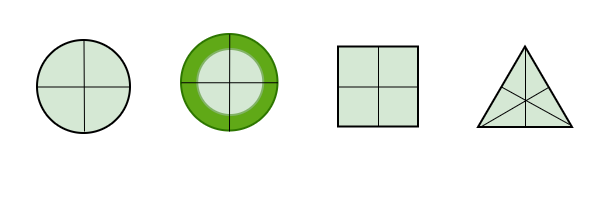Notice, for a ring, its Centre of mass lies inside the ring, which means it is necessary that the Centre of mass of a body lies in the body itself.

### Finding the Centre of Mass

Now, it is clear that bodies that are uniform and symmetrical have their Centre of masses at their centroid. But for bodies that are not symmetrical and uniform, the answer is not that simple. The Centre of mass for such bodies can be anywhere. To work out the Centre of mass of a complex object. A weighted average of the locations of each mass of the body is taken.

Let’s say there is a body consisting of a set of masses “mi“, each at position ri, the location of the Centre of mass rcm is given by the formula below.

Mrcm = m1r1 + m2r2 + ….

⇒ rcmIn this case, M =, which is the total mass of the body.

The above technique uses vector arithmetic. To avoid vector arithmetic, we can find out the Centre of mass of the body along the x-axis and y-axis respectively. Formulas for this case are given below:

xcmycmCentre of Gravity

Usually, gravity is assumed to be a uniform force acting on the body. The Centre of gravity is the point at which gravity is assumed to be acting on the body. The Centre of gravity is thus at the same place as the Centre of mass. In physics literature, the terms Centre of gravity and Centre of mass are used interchangeably. They mean the same thing.

Important Centre of Mass Formulas

Some systems occur more frequently in real life than others. While calculating the Centre of mass for such systems, the traditional method takes time. Certain Centre of mass formulas should be kept in mind while solving the questions related to the Centre of mass. These formulas help in simplifying the calculations.

1. System of two point masses

In such a system, COM lies closer to the heavier mass.m1r1 = m2r2

Distance of COM from mass m1Distance of COM from mass m22. Equilateral Triangle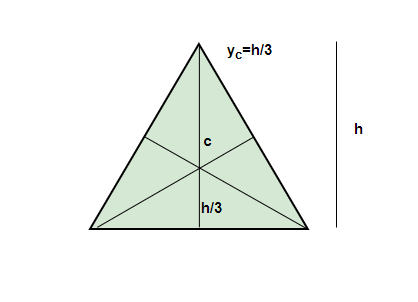The Centre of mass lies at the centroid, which is at the height of3. Semi-Circular Disk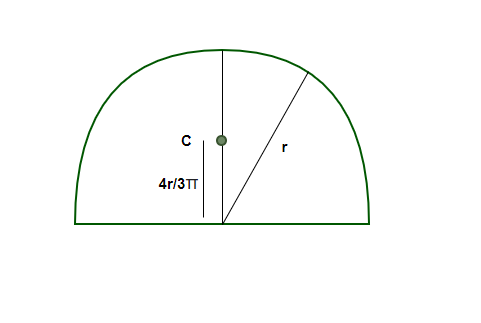yc =4. Semi-Circular ring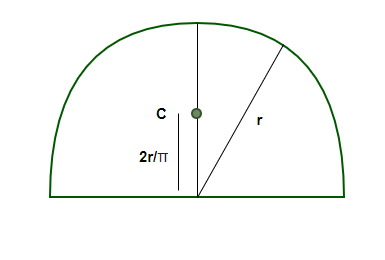yc =Let’s look at some sample problems

### Sample Problems

Question 1: Two point masses, m1 = 5Kg and m2 = 2Kg, are located at x = 2 m and x = 6 m respectively. Find the Centre of mass.

Solution:

The formula for the Centre of mass is given by,

xcmm1 = 5Kg, m2 = 2Kg and x = 2 m and x = 6 m.

M = m1 + m2

⇒ M = 5 + 2 = 7

xcm⇒ xcm⇒ xcm⇒ xcmQuestion 2: Twopoint masses, m1 = 5Kg and m2 = 2Kg, are located at y = 10m and y = -5 m respectively. Find the Centre of mass.

Solution:

The formula for the Centre of mass is given by,

ycmm1 = 5Kg, m2 = 2Kg and y = 10m and y = -5 m.

M = m1 + m2

⇒ M = 5 + 2 = 7

ycm⇒ ycm⇒ ycm⇒ ycmQuestion 3: Twopoint masses, m1 = 1Kg and m2 = 2Kg, are located at vector a = 6i + 4j and vector b = -5i + 2j respectively. Find the Centre of mass.

Solution:

The formula for the Centre of mass in the vector notation is given by,

rcmm1 = 1Kg, m2 = 2Kg and a = 6i + 4j, b = -5i + 2j

M = m1 + m2

⇒ M = 1 + 2 = 3

rcm⇒rcm⇒ rcm⇒ rcm⇒ rcmQuestion 4: Twopoint masses, m1 = 4Kg and m2 = 2Kg, are located at vector a = i + j and vector b = -i + j respectively. Find the Centre of mass.

Solution:

The formula for the Centre of mass in the vector notation is given by,

rcmm1 = 4Kg, m2 = 2Kg and a = i + j, b = -i + j

M = m1 + m2

⇒ M = 4 + 2 = 6

rcm⇒rcm⇒ rcm⇒ rcm⇒ rcmQuestion 5: A disk of radius R/2 is removed from a bigger disk of mass M of radius R. Find the Centre of mass.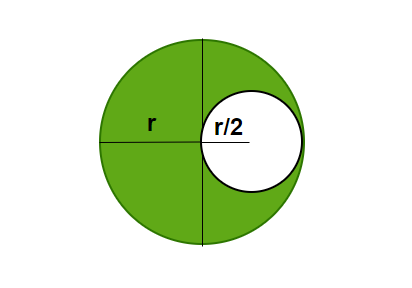Solution:

Since the density of the disk is uniform, the weight is uniformly distributed over all the area.

Mass “m” of the removed disk =Figure

The figure shows the center of masses of the remaining portion and the removed portion. Notice that if both of these are taken together, the center of mass should lie at the Centre. Let the distance of the center of mass of the remaining portion be “x”.Question 6: A disk of radius R/4 is removed from a bigger disk of mass M of radius R, in the same manner as the previous figure. Find the Centre of mass.

Solution:

Since the density of the disk is uniform, the weight is uniformly distributed over all the area.

Mass “m” of the removed disk =The figure shows the center of masses of the remaining portion and the removed portion. Notice that if both of these are taken together, the center of mass should lie at the Centre. Let the distance of the center of mass of the remaining portion be “x”.My Personal Notes arrow_drop_up